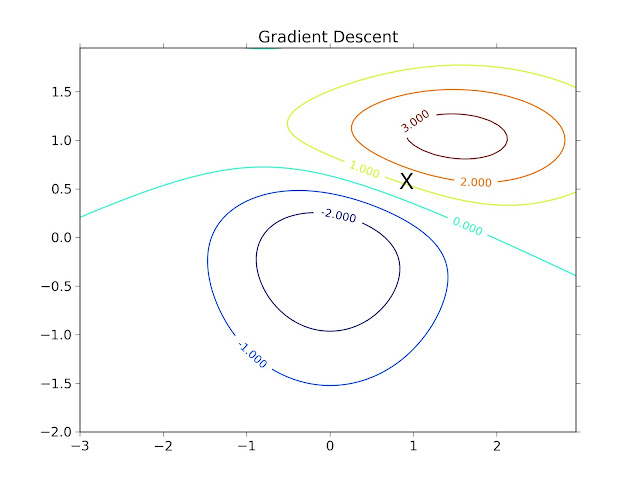### [ML, Python] Gradient Descent Algorithm (revision 2)

Linear regression, 基本上高中數學課本就已經有教過了

x就是那些點 (input), 那 hθ(x) 就是那條可能的線

θ其實就是以前國中高中常看到的 y = ax + b 的 a 跟 b

Data Point:

```1.1 3.17
2 3.81
2.2 4.15
3.1 3.75
3.5 4.15
4.1 4.25
4.7 4.36
5.3 4.56
5.8 5.06
6.4 5.36
```

summation裡面的就是剛剛我們做的, 把點帶入, 減去真正的y

(當然原因還有很多, 還有點連不連續問題...etc)

(注意: 並不是cost function都長這樣, 這只是linear regression的cost設計成這樣)

1. 初始話θ (通常都先從0開始)

2. 開始找θ, 而且希望會reduce J(θ), 希望可以找到夠小的值(深褐色是高點, 深藍色是低點, 其餘在中間)

Coursera上面有特別提醒一件事情, 在實作的時候, 要注意, 更新這兩個要同時更新

Coursera上補充了一點, 很有趣的是, linear regression的cost function, 在設計上, 會造成ball shape function, 也稱convex function, 會讓本來local minimum的gradient descent在這個case下, 變成global minimum (原因請看optimization class的convex相關內容)

Normal Equation wiki: http://en.wikipedia.org/wiki/Linear_least_squares_(mathematics)

Implementation

```from numpy import *
import matplotlib.pyplot as plt
```

```data = matrix(genfromtxt('aba67', dtype=float, delimiter=','))
X = data[:, 0]
y = data[:, 1]
m = size(y)
```

2. Compute Cost

```def computeCost(X, y, theta):
m = size(y);
return (1.0 / (2.0 * m)) * sum(power(X * theta - y, 2));

oneX = concatenate((ones((m,1)), data[:,0]), axis = 1)
theta = zeros((2,1))

print computeCost(oneX, y, theta)
```

```
def gradientDescent(X, y, theta, alpha, num_iters):
m = size(y)
n = size(theta)
tmp = zeros((n,1))
for iter in xrange(1, num_iters + 1):
for initer in xrange(0, n):
tmp[initer] = theta[initer] - alpha * (1.0 / m) * sum(transpose(X * theta - y) * X[:,initer])
theta = tmp
return theta

iterations = 3000
alpha = 0.1

theta = gradientDescent(oneX, y, theta, alpha, iterations)
```

```from numpy import *
import matplotlib.pyplot as plt

def computeCost(X, y, theta):
m = size(y);
return (1.0 / (2.0 * m)) * sum(power(X * theta - y, 2));

def gradientDescent(X, y, theta, alpha, num_iters):
m = size(y)
n = size(theta)
tmp = zeros((n,1))
for iter in xrange(1, num_iters + 1):
for initer in xrange(0, n):
tmp[initer] = theta[initer] - alpha * (1.0 / m) * sum(transpose(X * theta - y) * X[:,initer])
theta = tmp
return theta

if __name__ == '__main__':
data = matrix(genfromtxt('aba67', dtype=float, delimiter=','))

X = data[:, 0]
y = data[:, 1]
m = size(y)

oneX = concatenate((ones((m,1)), data[:,0]), axis = 1)
theta = zeros((2,1))

print computeCost(oneX, y, theta)

iterations = 3000
alpha = 0.1

theta = gradientDescent(oneX, y, theta, alpha, iterations)

plt.plot(X, y, 'rx')
plt.plot(oneX[:,1], oneX * theta, '-')
plt.xlabel('Shucked weight')
plt.ylabel('Viscera weight')
plt.show()
```

```theta = linalg.pinv(transpose(oneX)*oneX)*transpose(oneX)*y;
```

θ in Gradient Descent: [-0.00212767, 0.56028958]

θ in Normal Equation: [-0.00240189, 0.56121222]

### 留言

1.Hi, 剛好看到HHtu的note,其實我也是剛好看到Coursera中ML的這部份,有寫問題想請教一下!

在更新theta0和theta1的時候要同時更新這裡我有看到,只是一直不太了解為什麼?

如果能幫忙回答真是萬分感謝:D

2.喔, 原因是因為他要更新這回的 theta 是根據上一回的 theta

假設沒有同時更新

實作上, 如果你先更新了 theta_0, 則要更新 theta_1 的時候,

會使用到的是新的 theta_0 跟舊的 theta_1, 那算出來的東西就不太正確囉~

應該要的是用舊的 theta_0 跟 theta_1 來更新參數

1.了解了,謝謝您!

3.請問非線性也也可以使用這個嗎
遇到的是y=axe^bx 看了很多人都先轉成線性才去做 但是有沒有辦法直接做呢